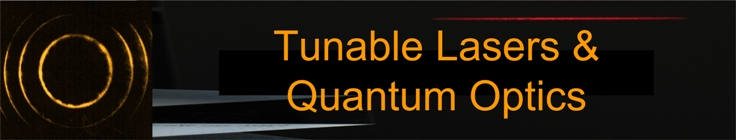## Generalized Refraction Equations

In 1997 from the generalized interferometric equation, derived from first principles using Dirac's quantum notation, a generalized refraction equation was derived. Thus, optics was described in a coherent and hierarchical order from interference, to diffraction, to refraction, and to reflection. In 2006, this approach was extended to provide generalized refraction equations for positive and negative refraction. Generalized refraction equations are also known as generalized Snell's laws.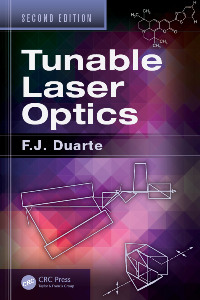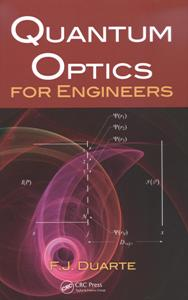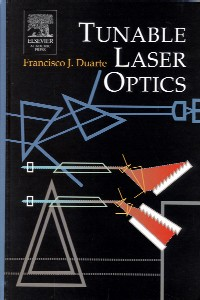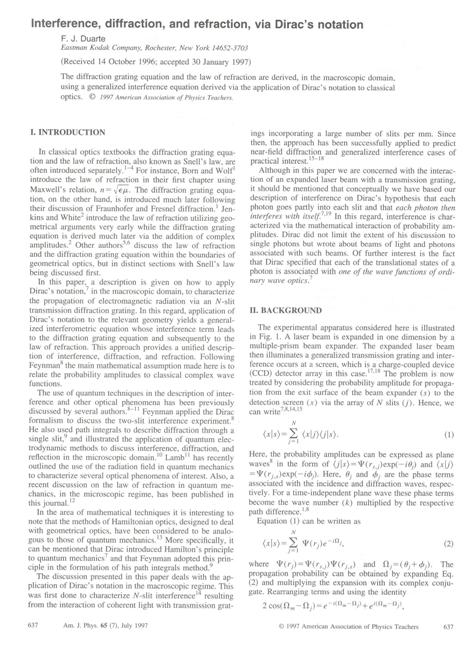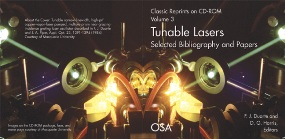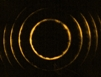First published on the 1st of March, 2007; Updated on the 4th of April, 2017.